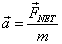# Regents Physics - Newton's 2nd Law of Motion

## Newton's 2nd Law

Newton's 2nd Law of Motion may be the most important principle in all of modern-day physics. It explains exactly how an object's velocity is changed by a net force. In words, Newton's 2nd Law states that "the acceleration of an object is directly proportional to the net force applied, and inversely proportional to the object's mass." In equation form:It's important to remember that both force and acceleration are vectors. Therefore, the direction of the acceleration, or the change in velocity, will be in the same direction as the net force.

You can also look at this equation from the opposite perspective. A change in an object's velocity, also known as acceleration, will produce a net force. This is the form in which Newton's 2nd Law if written on your reference table:## Problem Solving Strategies

You can solve many dynamics problems using the same basic steps:

1. Draw a free body diagram.
2. For any forces that don't line up with the x- or y-axes, break those forces up into
components that do lie on the x- or y-axis.
3. Write expressions for the net force in x- and y- directions. Set the net force equal to ma, since Newton's 2nd Law tells us that F=ma.
4. Solve the resulting equations.

Let's take a look at a sample problem:

Of course, everything we've already learned about kinematics still applies, and can be applied to Dynamics problems as well.

## Static EquilibriumThe special situation in which the net force on an object turns out to be zero, which we call static equilibrium, tells us immediately that the object isn't accelerating. If the object is moving with some velocity, it will remain moving with that exact same velocity. If the object is at rest, it will remain at rest. Sounds familiar, doesn't it? This is a restatement of Newton's 1st Law of Motion, the Law of Inertia. So in reality, Newton's 1st Law is just a special case of Newton's 2nd Law, describing static equilibrium conditions! Consider the situation of a tug-of-war... if both participants are pulling with tremendous force, but the force is balanced, there is no acceleration -- a great example of static equilibrium.

Static equilibrium conditions are so widespread that knowing how to explore and analyze these conditions is a key stepping stone to understanding more complicated situations.One common analysis question involves finding the equilibrant force given a free body diagram on an object. The equilibrant is a single force vector that you add to the unbalanced forces on an object in order to bring it into static equilibrium. For example, if you are given a force vector of 10N north and 10N east, and asked to find the equilibrant, you're really being asked to find a force that will offset the two given forces, bringing the object into static equilibrium.To find the equilibrant, you must first find the net force being applied to the object. To do this, we revisit our vector math and add up the two vectors by first lining them up tip to tail, then drawing a straight line from the starting point of the first vector to the ending point of the last vector. The magnitude of this vector can be found from the Pythagorean Theorem.Finally, to find the equilibrant vector, we need to add a single vector to the diagram that will give a net force of 0. If our total net force is currently 14N NE, then the vector that should bring this back into equilibrium, the equilibrant, must be the opposite of 14N NE, or a vector with magnitude 14N in the SW direction.

Another common analysis question involves being asked whether three vectors could be arranged to provide a static equilibrium situation. For example:

"Can the three vectors 5N, 8N, and 12N be in equilibrium?"

To solve a question like this, we'll walk through three easy steps.

1. Add the first two vectors: 5N + 8N = 13N
2. Subtract the first two vectors: 8N-5N = 3N
3. If the remaining vector falls between your answers to #1 and #2, you can have an equilibrium situation if the vectors are lined up properly.

In this case, then, 5N, 8N, and 12N can be in equilibrium. Test yourself by proving that the force vectors 5N, 6N, and 12N cannot be in equilibrium. Let's try a problem from the June 2006 Regents Physics Exam: